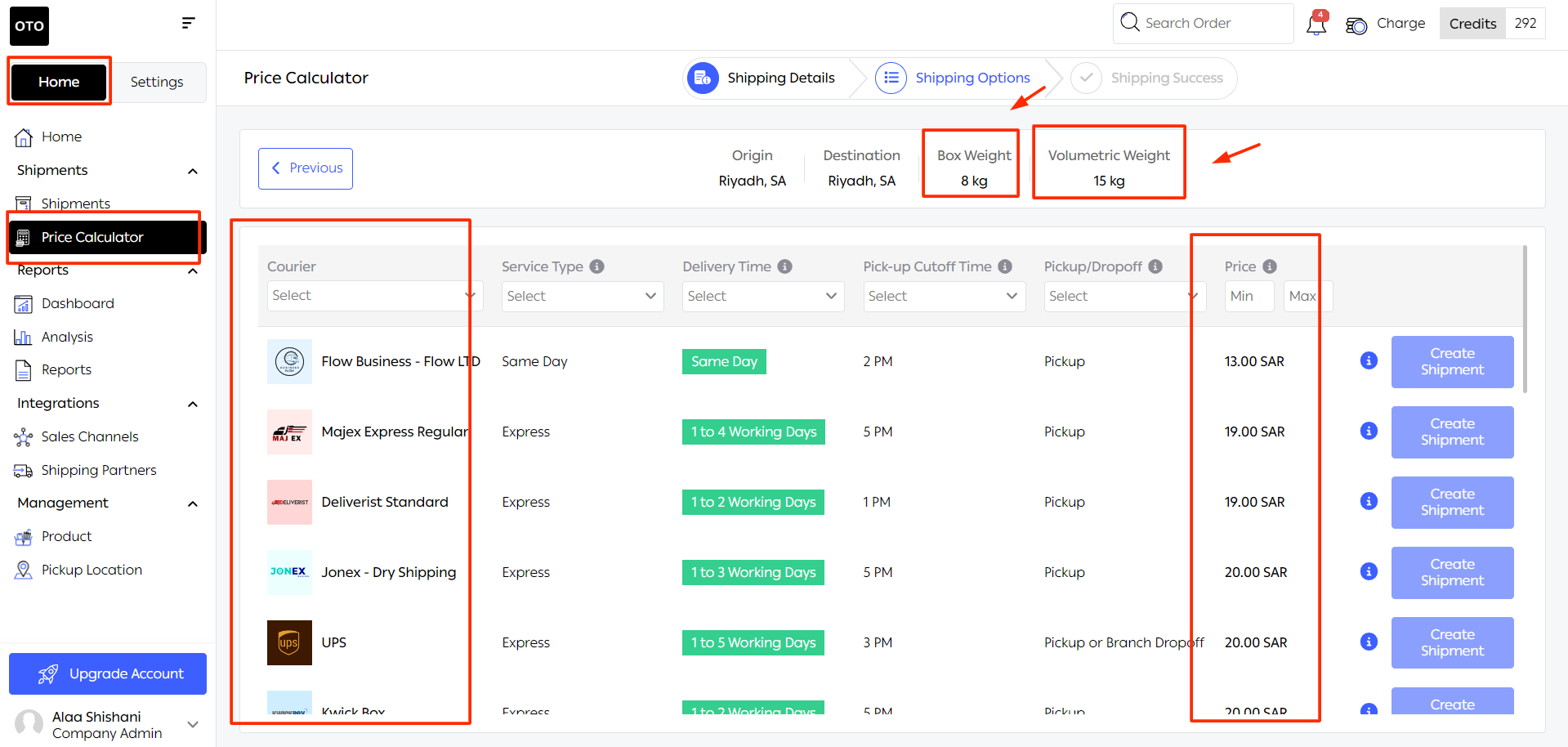# Difference between Actual Weight and volumetric Weight

Created by Malek Al Najdawi, Modified on Thu, 13 Jul 2023 at 10:22 AM by Malek Al Najdawi

In the world of shipping and logistics, understanding the difference between actual weight and volumetric weight is of utmost importance. These two terms play a crucial role in calculating shipping costs, especially when dealing with lightweight but large items.

To illustrate the difference, let's use the example of cotton and iron.

If we compare two shipments, one consisting of 100 kilograms of cotton and the other of 100 kilograms of iron, we might think that they have the same actual weight, right?

However, in reality, we would find that the volumetric weight differs for each of them, as the volume of cotton would be larger than that of iron.

Now, let's explain the meaning of each term individually with illustrative examples:

Actual Weight:

Actual weight refers to the true weight of an object, usually measured in kilograms. It is the physical weight of the item as measured on a scale. Determining the actual weight is straightforward since it is a direct measure of the object's mass.

For example, if you have a shipment weighing 5 kilograms, the actual weight of the shipment is 5 kilograms. Simple, isn't it? Now, let's move on to volumetric weight.

Volumetric Weight:

Volumetric weight takes into account the dimensions or volume of an object. It is a calculated weight based on the amount of space the object occupies during transportation, which significantly affects shipping costs.

For example, volumetric weight becomes particularly important when shipping lightweight but large items that occupy more space than their actual weight would suggest. Think of items like pillows or inflatable toys, which are light but have substantial dimensions.

How to calculate Volumetric Weight?

To calculate volumetric weight, you need to multiply the length, width, and height of the package (in centimeters or inches) and divide the result by the predefined volumetric factor.

Important note: The volumetric factor may vary between shipping companies, but most companies use 5000 or 4000 as the standard conversion factor for land freight and 6000 for air freight.

To calculate volumetric weight, follow these simple steps:

1. Measure the dimensions of the package (length, width, and height).2. Calculate the volume of the package by multiplying the length, width, and height.

3. Divide the volume by the volumetric factor.

As mentioned earlier, it is typically 5000 or 4000 for land freight and 6000 for air freight.

4. Round the result to the nearest whole number.

Let's simplify it with a practical example:

Suppose you have a shipping box with an actual weight of 8 kilograms. How do you measure its volumetric weight?

Let's assume the box has the following dimensions:

Length: 50 cm

Width: 40 cm

Height: 30 cm

We'll use the volumetric factor 4000 to calculate the volumetric weight.

Calculate the volume of the package:

Volume = Length x Width x Height

Volume = 50 cm x 40 cm x 30 cm

Volume = 60,000 cm³

Convert the volume to kilograms using the volumetric factor:

Volumetric Weight = Volume / Volumetric Factor

Volumetric Weight = 60,000 cm³ / 4000

Volumetric Weight = 15 kilograms

So, in this case, the volumetric weight is 15 kilograms, while the actual weight is 8 kilograms.

To determine the weight used for shipping charges, compare the actual weight and the volumetric weight, and select the higher value. In this example, the chargeable weight would be 15 kilograms as it is higher than the actual weight of 8 kilograms.

OTO Price Calculator

At OTO, you can easily find out the volumetric weight along with the prices of the shipping options available through the price calculator feature. Let's put the previous example with the same details on OTO's price calculator and see the shipping options available.